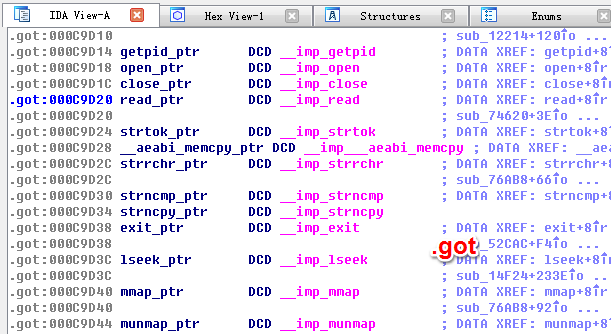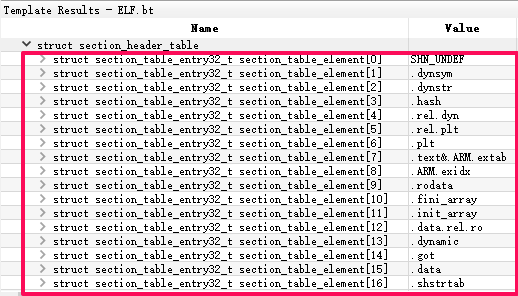# ELF的内存分布

``````{ [0x0h]elf(.so)
{ [0x0h]ehdr
} [0x33h]ehdr

{ [0x34h]PT_PHDR / phdr
} [0x133h]PT_PHDR / phdr

{ [0x134h].interp / PT_INERP
} [0x146h].interp / PT_INERP

/* gap size: 1h  */
{ [0x148h].dynsym / DT_SYMTAB
} [0x607h].dynsym / DT_SYMTAB

{ [0x608h].dynstr / DT_STRTAB
} [0xbdah].dynstr / DT_STRTAB

/* gap size: 1h  */
{ [0xbdch].hash / DT_HASH
} [0xe1fh].hash / DT_HASH

{ [0xe20h].rel.dyn / DT_REL
} [0xe67h].rel.dyn / DT_REL

{ [0xe68h].rel.plt / DT_JMPREL
} [0xf1fh].rel.plt / DT_JMPREL

{ [0xf20h].plt
} [0x1047h].plt

{ [0x1048h].text
} [0x2b23h].text

{ [0x2b24h].ARM.extab
} [0x2b8fh].ARM.extab

{ [0x2b90h].ARM.exidx
} [0x2cbfh].ARM.exidx

{ [0x2cc0h].rodata
} [0x2d2fh].rodata

/* gap size: 113ch  */
{ [0x3e6ch]PT_UNK_70000001h
{ [0x3e6ch].fini_array / DT_FINI_ARRAY
} [0x3e73h].fini_array / DT_FINI_ARRAY

{ [0x3e74h].init_array / DT_INIT_ARRAY
} [0x3e77h].init_array / DT_INIT_ARRAY

{ [0x3e78h].dynamic / PT_DYNAMIC
} [0x3f77h].dynamic / PT_DYNAMIC

{ [0x3f78h].got
{ [0x3f98h]DT_PLTGOT
} [0x3fffh]DT_PLTGOT
} [0x3fffh].got
} [0x3fffh]PT_UNK_70000001h

{ [0x4000h].data
} [0x4003h].data
} [0x4003h]elf(.so)
``````

# 案例分析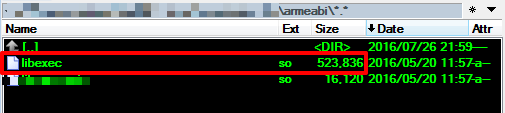section只有一个有效项`.strtab`: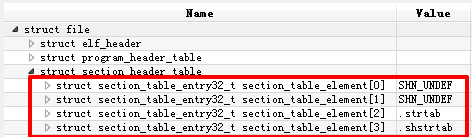dump后section已经丢失: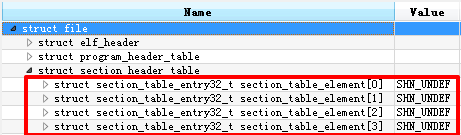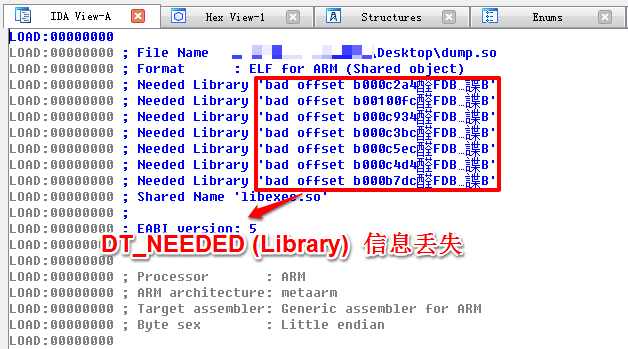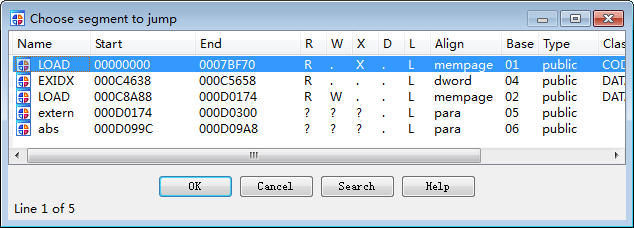# SECTION重建

## 通过PT_DYNAMIC重建

.hash / DT_HASH

.rel.dyn / DT_REL

.interp / PT_INERP

.dynsym / DT_SYMTAB

.dynstr / DT_STRTAB

.rel.plt / DT_JMPREL

.dynamic / PT_DYNAMIC

.fini_array / DT_FINI_ARRAY

.init_array / DT_INIT_ARRAY

section name dynamic type size
.hash DT_HASH (nbucket+nchain)*4+8
.rel.dyn DT_REL DT_RELSZ
.rel.plt DT_JMPREL DT_PLTSZ
.interp PT_INERP strlen(ptr)+1
.dynsym DT_SYMTAB nchain*sizeof(Elf32_Sym)
.dynstr DT_STRTAB DT_STRSZ
.dynamic PT_DYNAMIC PT_DYNAMIC->p_filesz
.fini_array DT_FINI_ARRAY DT_FINI_ARRAYSZ
.init_array DT_INIT_ARRAY DT_INIT_ARRAYSZ

## 通过固有位置重建

### .plt的重建

#### .plt位置

``````    { [0xe68h].rel.plt / DT_JMPREL
} [0xf1fh].rel.plt / DT_JMPREL

{ [0xf20h].plt
} [0x1047h].plt
``````

#### .plt大小

.plt头部会有0x14个固定作用的数据(0x10指令+0x4偏移)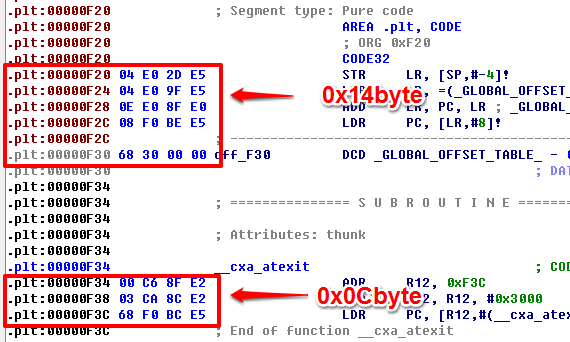### .got的重建

#### .got位置

.got项可以通过DT_REL与DT_JMPREL中的Elf32_Rel::r_offset来依次遍历得到, 通过其中的最小和最大地址来确定.got的位置和大小.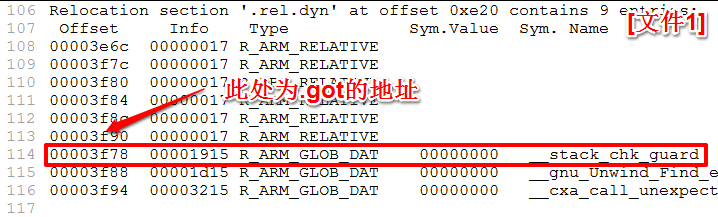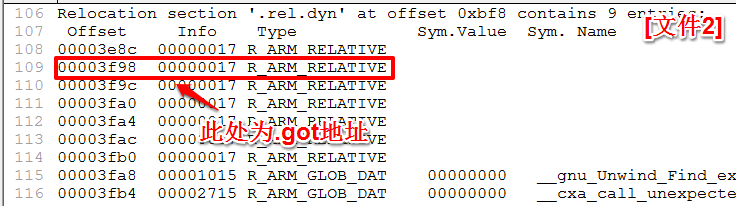``````      { [0x3e78h].dynamic / PT_DYNAMIC
} [0x3f77h].dynamic / PT_DYNAMIC

{ [0x3f78h].got
{ [0x3f98h]DT_PLTGOT
} [0x3fffh]DT_PLTGOT
} [0x3fffh].got
} [0x3fffh]PT_UNK_70000001h
``````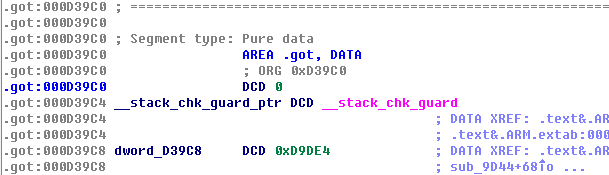### .data的重建

#### .data位置

``````      } [0x3fffh].got
} [0x3fffh]PT_UNK_70000001h

{ [0x4000h].data
} [0x4003h].data
``````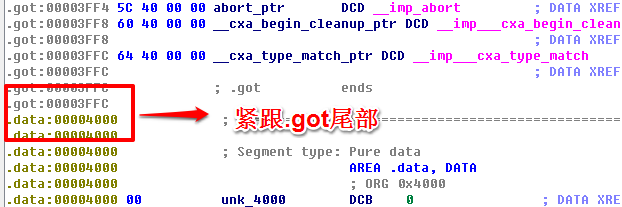### .rodata的重建

#### .rodata的位置

``````    { [0x2b90h].ARM.exidx
} [0x2cbfh].ARM.exidx

{ [0x2cc0h].rodata
} [0x2d2fh].rodata
``````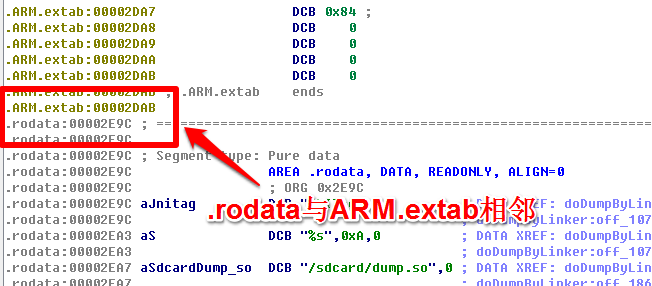# DT_NEEDED的修复

``````struct soinfo {
public:
char name[SOINFO_NAME_LEN];
/*...*/
}
``````

# 最终效果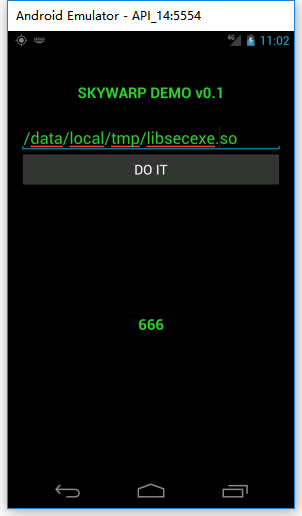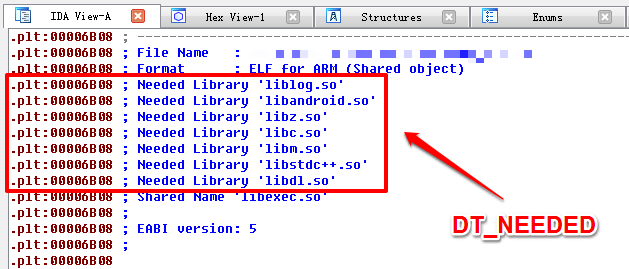.plt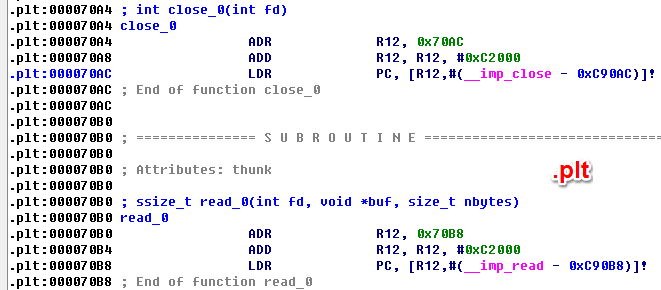.got